Practice Interpreting Graphs Terms of Use    Contact Person: Donna RobertsDirections: Solve the following problems dealing with statistical graphs.

1.  Questions pertain to the histogram shown below.
(a) How many students are in the Fencing Club?
 Choose: 17 18 19 20

(b) How many students' heights are greater than or equal to 66 inches tall?
 Choose: 5 6 7 11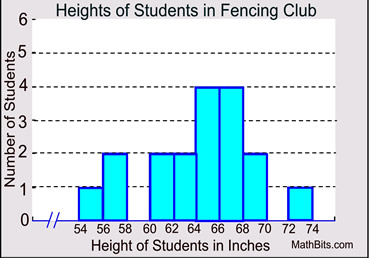(c)
What percentage of the students have a height greater than or equal to 60 inches but less than 66 inches, to the nearest tenth of a percent?

 Choose: 44.4% 55.2% 66.7% 80.0%

(d)
In which interval, will you find the median student height, h?

Choose:
 60 ≤ h < 62 62 ≤ h < 64 64 ≤ h < 66 66 ≤ h < 68

2.  The box and whisker plots below represent the number of points scored in each game for the 2013 fall football season for two area high school teams.
(a) In general, which team scored more points per game?
 Choose: Team A Team B

(b)
Which team's data shows more variability in the points scored?

 Choose: Team A Team B

(c)
What is the difference between the median points scored by these two teams?
 Choose: 4 5 8 9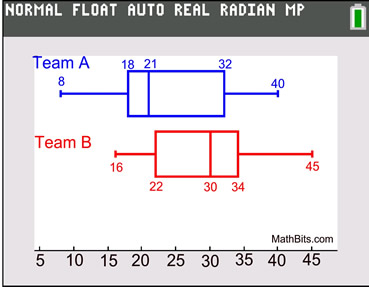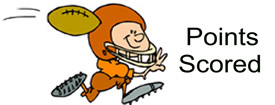3.  In the same morning, fifteen people spend money on \$5 lottery tickets at a local gas station. The dot plot shows the amount spent by each customer on the tickets.
(a) What is the median of the dollars spent?
 Choose: \$20 \$30 \$40 \$45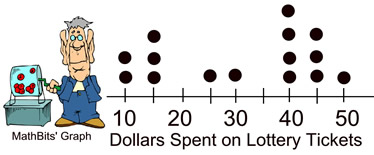(b) What is the mean (average) of the dollars spent?
 Choose: \$20 \$30 \$31 \$40

(c)
What monetary value represents the first quartile of this data?

 Choose: \$15 \$25 \$40 \$45

(d)
What is the interquartile range?

 Choose: 15 25 30 45

(e)
What is the population standard deviation for this data
(to the nearest hundredth)?
 Choose: 12.46 13.96 14.05 14.54

4.  The box and whisker plots shown below represent monthly temperatures (º F) for three cities.

(a)
Which city has temperatures most evenly spread over the greatest range?

 Choose: A B C

(b)
Which city has a potential outlier among its data?

 Choose: A B C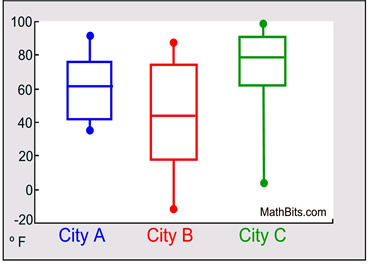(c)
Which city has temperatures consistently above freezing (32º F)?

 Choose: A B C

5.  Examine the histograms shown below. Complete the chart below by choosing the graph that best shows the stated result.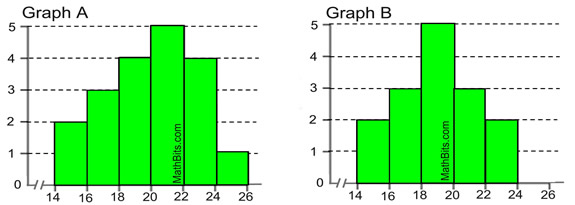Graph A
Graph B
Both Graphs
Larger Median
Symmetric Graph
Smaller Range
Larger Data Count
Interval Scale of 2
Unimodal

6.  Two game players have been recording their best scores each day during a one week video tournament. Based on the box plots shown below, determine if the following statements are true or false.

(a)
The median score of
Player A is greater than the median score of Player B.
 Choose: True False

(b)
The highest score was obtained by
Player B.
 Choose: True False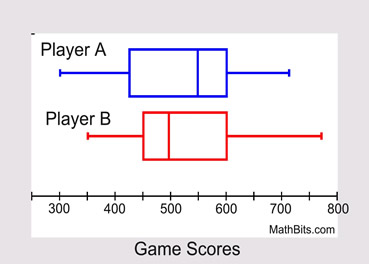(c)
The interquartile range of the scores of
Player B is less than the interquartile range of the scores of Player A.
 Choose: True False

7.  Examine the dot plots shown below. Complete the chart below by comparing the two plots.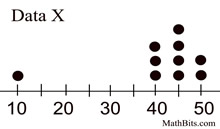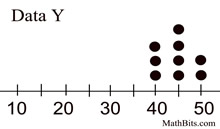Larger in
Data X
Same in both
data sets
Larger in
Data Y
Median
Range
Mean
Standard Deviation
Mode
Maximum